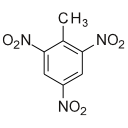Subsections

## structure.atomic_fluctuations### Synopsis

Create an interatomic distance fluctuation correlation matrix.

### Defaults

structure.atomic_fluctuations(pipes=None, models=None, molecules=None, atom_id=None, measure=`distance', file=None, format=`text', dir=None, force=False)

### Keyword arguments

pipes: The data pipes to generate the interatomic distance fluctuation correlation matrix for.

models: The list of models for each data pipe to generate the interatomic distance fluctuation correlation matrix for. The number of elements must match the pipes argument. If no models are given, then all will be used.

molecules: The list of molecules for each data pipe to generate the interatomic distance fluctuation correlation matrix for. This allows differently named molecules in the same or different data pipes to be superimposed. The number of elements must match the pipes argument. If no molecules are given, then all will be used.

atom_id: The atom identification string of the coordinates of interest. This can be used to restrict the correlation matrix to one atom per residue, for example.

measure: The type of fluctuation to investigate. This allows for both interatomic distance and vector angle fluctuations to be calculated.

file: The name of the text file to create.

format: The output format. For all formats other than the text file, a second file will be created with the same name as the text file but with the appropriate file extension added.

dir: The directory to save the file to.

force: A flag which if set to True will cause any pre-existing files to be overwritten.

### Description

This is used to visualise the interatomic fluctuations between different structures. By setting the measure argument, different categories of fluctuations can seen:

`distance' -
The interatomic distance fluctuations is the default option. The corrected sample standard deviation (SD) is calculated for the distances between all atom pairs, resulting in a pairwise matrix of SD values. This is frame independent and hence is superimposition independent.
`angle' -
The interatomic vector angle fluctuations. The corrected sample standard deviation (SD) is calculated for the angles between the inter atom vectors all atom pairs to an average vector. This also produces a pairwise matrix of SD values.
`parallax shift' -
The interatomic parallax shift fluctuations. The corrected sample standard deviation (SD) is calculated for the parallax shift between the inter atom vectors all atom pairs to an average vector. This also produces a pairwise matrix of SD values. The parallax shift is calculated as the dot product of the interatomic vector and the unit average vector, times the unit average vector. It is a frame and superimposition dependent measure close to orthogonal to the interatomic distance fluctuations. It is similar to the angle measure however, importantly, it is independent of the distance between the two atoms.

For the output file, the currently supported formats are:

`text' -
This is the default value and will result in a single text file being created.
`gnuplot' -
This will create a both a text file with the data and a script for visualising the correlation matrix using gnuplot. The script will have the same name as the text file, however the file extension will be changed to *.gnu.

Support for multiple structures is provided by the data pipes, model numbers and molecule names arguments. Each data pipe, model and molecule combination will be treated as a separate structure. As only atomic coordinates with the same residue name and number and atom name will be assembled, structures with slightly different atomic structures can be compared. If the list of models is not supplied, then all models of all data pipes will be used. If the optional molecules list is supplied, each molecule in the list will be considered as a separate structure for comparison between each other.

The atom ID string, which uses the same notation as the spin ID, can be used to restrict the coordinates compared to a subset of molecules, residues, or atoms. For example to only use backbone heavy atoms in a protein, set the atom ID to `@N,C,CA,O', assuming those are the names of the atoms in the 3D structural file.

### Prompt examples

To create the interatomic distance fluctuation correlation matrix for the models 1, 3, and 5, type:

```[numbers=none]
relax> structure.atomic_fluctuations(models=[[1, 3, 5]], file='atomic_fluctuation_matrix')
```

To create the interatomic distance fluctuation correlation matrix for the molecules `A', `B', `C', and `D', type:

```[numbers=none]
relax> structure.atomic_fluctuations(molecules=[['A', 'B', 'C', 'D']], file='atomic_fluctuation_matrix')
```

The relax user manual (PDF), created 2020-08-26.